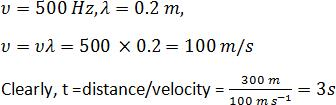Courses

# Sound - Practice Test, Class 9 Science

## 30 Questions MCQ Test | Sound - Practice Test, Class 9 Science

Description
This mock test of Sound - Practice Test, Class 9 Science for Class 9 helps you for every Class 9 entrance exam. This contains 30 Multiple Choice Questions for Class 9 Sound - Practice Test, Class 9 Science (mcq) to study with solutions a complete question bank. The solved questions answers in this Sound - Practice Test, Class 9 Science quiz give you a good mix of easy questions and tough questions. Class 9 students definitely take this Sound - Practice Test, Class 9 Science exercise for a better result in the exam. You can find other Sound - Practice Test, Class 9 Science extra questions, long questions & short questions for Class 9 on EduRev as well by searching above.
QUESTION: 1

### The time period of a vibrating body is 0.05 s. The frequency of waves it emits is?

Solution: Frequency = 1/time period
frequency (nu) = 1/0.05
multiply 1 and 0.05 by 100 to take out the decimal .
you will get 100/ 5= 20 .
i.e frequency = 20 Hz
QUESTION: 2

Solution:
QUESTION: 3

### If a vibrator strikes the water 10 times in one second, then the frequency of wave is _________.

Solution:
The amount of wave cycles a second is equivalent to the Frequency. There are ten wave cycles is one second.
The unit of frequency is now Hz.
1 vibration=1 hz,then
10 vibrations=1*10
=10Hz is the solution.

QUESTION: 4
The method of detecting the presence, position and direction of motion of distant objects by reflecting a beam of sound waves is known as _____.
Solution:
QUESTION: 5
The minimum distance between the source and the reflector, so that an echo is heard is approximately equal to ______.
Solution:
QUESTION: 6
Which of the following will remain unchanged when a sound wave travels in air or in water?
Solution:
QUESTION: 7

Unit of wavelength is __________.

Solution:
QUESTION: 8
SI Unit of time period is __________.
Solution:
QUESTION: 9
Which of the following quantities is transferred during wave propagation?
Solution:
QUESTION: 10
When a wave travels through a medium ______.
Solution:
QUESTION: 11
Sound waves do not travel through?
Solution:
QUESTION: 12
The amplitude of a wave is ______.
Solution:
QUESTION: 13
The persistence of audible sound due to the successive reflections from the surrounding objects even after the source has stopped to produce that sound is called _________.
Solution:
QUESTION: 14
The unit of quantity on which pitch of the sound depends is :
Solution: It is because  pitch is that characteristic of sound that depends on the frequency received by a human ear.
and as we know that the S.I. unit of frequency is hertz therefore the unit of quantity on which pitch of the sound depends is hertz.
QUESTION: 15
Which of the following is not a characteristic of a musical sound?
Solution:
QUESTION: 16
The vibrations or the pressure variations inside the inner ear are converted into electrical signals by the_________.
Solution:
QUESTION: 17
The technique used by bats to find their way or to locate food is _______.
Solution:
QUESTION: 18
What is the velocity of sound in water at room temperature?
Solution: The temperature of water is greater than the air, in room temp. and the sound travels faster in high temp. therefore the velocity of sound in water is 1500 and in air is 340
QUESTION: 19
The speed of sound in medium depends upon?
Solution:
QUESTION: 20
A source of frequency of 500 Hz emits waves of wavelength 0.4 m, how long does the waves take to travel 600 m?
Solution:QUESTION: 21
The frequency of a wave travelling at a speed of 500 ms-1 is 25 Hz. Its time period will be ______.
Solution:
QUESTION: 22
The physical quantity, which oscillates in most waves, is?
Solution:
QUESTION: 23
Vibrations inside the ear are amplified by the three bones namely the _________ in the middle ear.
Solution:
QUESTION: 24
Bats detect the obstacles in their path by receiving the reflected ______.
Solution:
QUESTION: 25
When sound travels through air, the air particles ______.
Solution:
QUESTION: 26
Sound waves are :
Solution:
QUESTION: 27
A sound source sends waves of 400 Hz. It produces waves of wavelength 2.5 m. The velocity of sound waves is :
Solution:
QUESTION: 28
An example for mechanical wave.
Solution: A mechanical wave is a wave that is not capable of transmitting its energy through a vacuum. Mechanical waves require a medium in order to transport their energy from one location to another. A sound wave is an example of a mechanical wave. Sound wavesare incapable of traveling through a vacuum.
QUESTION: 29

An ultrasonic wave is sent from a ship towards the bottom of the sea. It is found that the time interval between the sending and receiving of the wave is 1.6 s. What is the depth of the sea, if the velocity of sound in the seawater is 1400 m/s?

Solution:
Total distance travelled by the ultrasonic waves=velocity of the ultrasonic waves*Total time taken by the ultrasonic waves
Therefore,
2*Depth of the sea=1400m/s*1.6s
Depth of sea=1120m
QUESTION: 30
The frequency which is not audible to the human ear is :
Solution: